# 前情提要——二叉树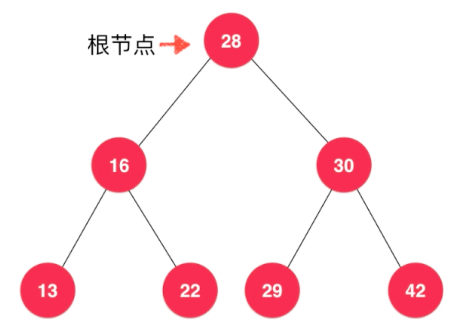#### Create a BST

package Tree.BST;

public class BST<E extends Comparable<E>> {
/**
* Binary Search Tree
*/

private class Node {
public E e;
public Node left, right;

public Node(E e) {
this.e = e;
left = right = null;
}
}

private Node root;
private int size;

public BST() {
root = null;
size = 0;
}

public int size(){
return size;
}

public boolean isEmpty(){
return size == 0;
}
}



#### Insert an element

    public void add(E e) {
}

private Node add(Node node, E e) {
if (node == null) {
size++;
return new Node(e);
}
if (e.compareTo(node.e) < 0) {
} else {
}
return node;
}


#### Select an element

···
public boolean contains(E e) {
return contains(root, e);
}

public boolean contains(Node node, E e) {
if (node == null) {
return false;
} else if (e.equals(node.e)) {
return true;
} else if (e.compareTo(node.e) < 0) {
return contains(node.left, e);
} else {
return contains(node.right, e);
}
}


···

#### Traversal

public void preOrderNonRecur() {
Stack<Node> stack = new Stack<>();
stack.push(root);
while (!stack.isEmpty()) {
Node node = stack.pop();
System.out.print(node.e + " ");
if (node.right != null)
stack.push(node.right);
if (node.left != null)
stack.push(node.left);
}
System.out.println();
}




public void inOrderNonRecur() {
Stack<Node> stack = new Stack<>();
Node node = root;
while (node != null || !stack.isEmpty()) {
while (node != null) {
stack.push(node);
node = node.left;
}
if (!stack.isEmpty()) {
Node node1 = stack.pop();
System.out.print(node1.e + " ");
node = node1.right;
}
}
System.out.println();



public void lastOrderNonRecur() {
Stack<Node> stack = new Stack<>();
Node node = root;
while (node != null || !stack.isEmpty()) {
while (node != null) {
stack.push(node);
node = node.left;
}
if (!stack.isEmpty()) {
Node node1 = stack.peek();
if (!node1.isright) {
node1.isright = true;
node = node1.right;
} else {
node = stack.pop();
System.out.print(node.e + " ");
node = null;
}
}
}
System.out.println();
}



levelOrder(){
while (!nodes.isEmpty()){
Node node = nodes.remove();
System.out.print(node.e + " ");
if (node.left != null){
}
if (node.right != null){
}
}
System.out.println();
}



#### remove an specific element

    private Node remove(Node node, E e) {
if (node == null) {
return null;
} else if (e.compareTo(node.e) < 0) {
node.left = remove(node.left, e);
return node;
} else if (e.compareTo(node.e) > 0) {
node.right = remove(node.right, e);
return node;
} else{
if (node.left == null){
Node node1 = node.right;
node.right = null;
size--;
return node1;
}else if (node.right == null){
Node node1 = node.left;
node.left = null;
size--;
return node1;
}else {
Node successor = new Node(minimum(node.right).e);
successor.left = node.left;
successor.right = removeMin(node.right);
node.left = node.right = null;
return successor;
}
}

}


# 集合Set

#### Leecode804

Leecode804，摩斯码的判断：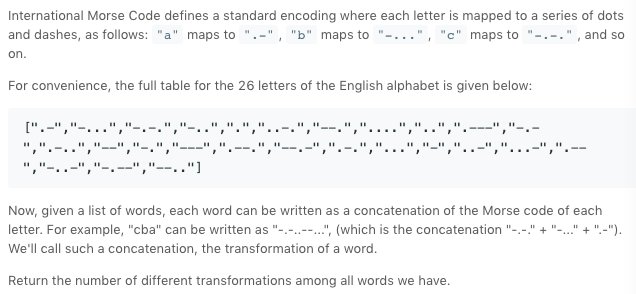class Solution {
public int uniqueMorseRepresentations(String[] words) {
String [] codes = {".-","-...","-.-.","-..",".","..-.","--.","....","..",".---","-.-",".-..","--","-.","---",".--.","--.-",".-.","...","-","..-","...-",".--","-..-","-.--","--.."};
TreeSet<String> set = new TreeSet<>();
for (String word : words){
StringBuilder stringBuilder = new StringBuilder();
for (int i = 0; i < word.length(); i++) {
stringBuilder.append(codes[word.charAt(i) - 'a']);
}
}
return set.size();
}
}


# 优先队列和堆

package Heap;

import Array.Array;

public class MaxHeap<E extends Comparable<E>> {
private Array<E> data;

public MaxHeap(int capacity) {
data = new Array<E>(capacity);
}

public MaxHeap() {
data = new Array<E>();
}

public int size() {
return data.getSize();
}

public boolean isEmpty() {
return data.isEmpty();
}

private int parent(int index) {
if (index == 0) {
throw new IllegalArgumentException("No parents when index equal zero!");
}
return (index - 1) / 2;
}

private int leftChild(int index) {
return index * 2 + 1;
}

private int rightChild(int index) {
return index * 2 + 2;
}

}



    private void shiftUp(int index) {
while (index > 0 && data.get(index).compareTo(data.get(parent(index))) > 0) {
data.swap(index, parent(index));
index = parent(index);
}
}


    private void shiftDown(int index){
while (leftChild(index) < data.getSize()){
int j = leftChild(index);
if (j + 1 < data.getSize() && data.get(j + 1).compareTo(data.get(j)) > 0){
j = rightChild(index);
}
if (data.get(index).compareTo(data.get(j)) >= 0){
break;
}
data.swap(index, j);
index = j;
}
}


    public E replace(E e) {
E max = data.get(0);
data.set(0, e);
shiftDown(0);
return max;
}


        for (int i = parent(this.data.getSize() - 1); i >= 0; i --){
shiftDown(i);
}


#### 优先队列347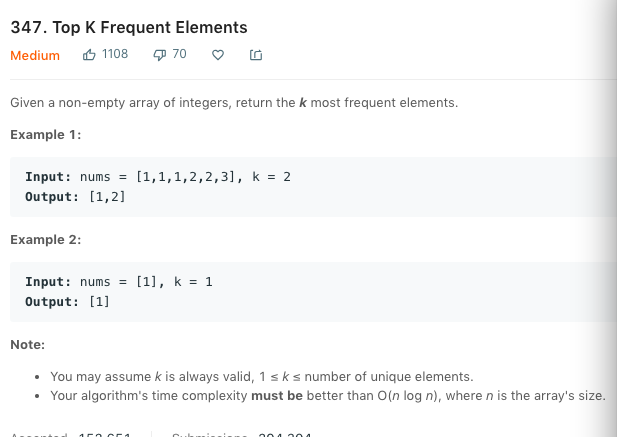package Queue.Leecode347;
import java.util.List;
import java.util.PriorityQueue;
import java.util.TreeMap;

class Solution {

private class Freq implements Comparable<Freq>{

int e, freq;

public Freq(int e, int freq){
this.e = e;
this.freq = freq;
}

@Override
public int compareTo(Freq another) {
if (this.freq < another.freq){
return -1;
}
else if (this.freq > another.freq){
return 1;
}else {
return 0;
}
}

}

public List<Integer> topKFrequent(int[] nums, int k) {
TreeMap<Integer, Integer> map = new TreeMap<>();
for (int n : nums) {
if (map.containsKey(n)) {
map.put(n, map.get(n) + 1);
} else {
map.put(n, 1);
}
}

PriorityQueue<Freq> priorityQueue = new PriorityQueue<>();
for(int key: map.keySet()){
if(priorityQueue.size() < k)
else if(map.get(key) > priorityQueue.peek().freq){
priorityQueue.remove();
}
}
while (!priorityQueue.isEmpty()){
}
}

public static void main(String[] args) {
int[] nums = {1,1,1,2,2,3};
Solution solution = new Solution();
System.out.println(solution.topKFrequent(nums, 2));
}

}


# 并查集

#### 并查集Quick Find


private int find(int p) {
if (p < 0 || p >= parent.length) {
throw new IllegalArgumentException("p is out of range!");
}
while (p != parent[p]) {
p = parent[p];
}
return p;
}

@Override
public int getSize() {
return parent.length;
}

@Override
public boolean isConnected(int p, int q) {
return find(p) == find(q);
}

@Override
public void unionElements(int p, int q) {
int pRoot = find(p);
int qRoot = find(q);
if (pRoot == qRoot){
return;
}
parent[pRoot] = qRoot;
}


#### 基于树的Quick union


private int find(int p) {
if (p < 0 || p >= parent.length) {
throw new IllegalArgumentException("p is out of range!");
}
while (p != parent[p]) {
p = parent[p];
}
return p;
}

@Override
public int getSize() {
return parent.length;
}

@Override
public boolean isConnected(int p, int q) {
return find(p) == find(q);
}

@Override
public void unionElements(int p, int q) {
int pRoot = find(p);
int qRoot = find(q);
if (pRoot == qRoot){
return;
}
parent[pRoot] = qRoot;
}


    @Override
public void unionElements(int p, int q) {
int pRoot = find(p);
int qRoot = find(q);
if (pRoot == qRoot) {
return;
}
if (sz[pRoot] < sz[qRoot]) {
parent[pRoot] = qRoot;
sz[qRoot] += sz[pRoot];
}else {
parent[qRoot] = pRoot;
sz[pRoot] += sz[qRoot];
}
}


    @Override
public void unionElements(int p, int q) {
int pRoot = find(p);
int qRoot = find(q);
if (pRoot == qRoot) {
return;
}
if (rank[pRoot] < rank[qRoot]){
parent[pRoot] = qRoot;
}else if (rank[pRoot] > rank[qRoot]){
parent[qRoot] = pRoot;
}else {
parent[qRoot] = pRoot;
rank[pRoot] += 1;
}


#### 路径压缩 Path Compression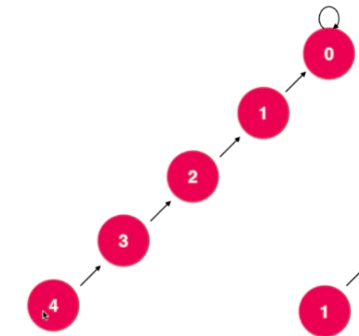private int find(int p) {
if (p < 0 || p >= parent.length) {
throw new IllegalArgumentException("p is out of range!");
}
while (p != parent[p]) {
parent[p] = parent[parent[p]];
p = parent[p];
}
return p;
}


# 哈希表HashTable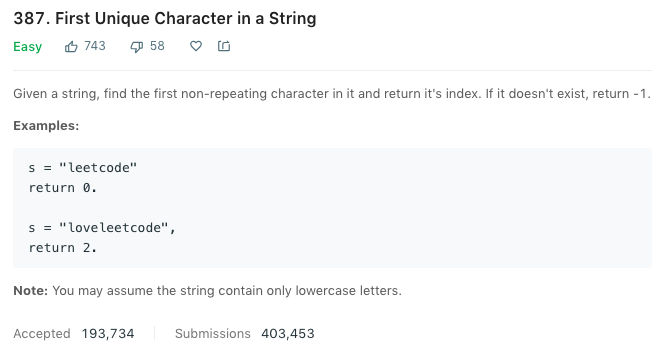class Solution {
public int firstUniqChar(String s) {
int[] freq = new int;
for (int i = 0; i < s.length(); i++) {
freq[s.charAt(i) - 'a']++;
}
for (int i = 0; i < s.length(); i++) {
if (freq[s.charAt(i) - 'a'] == 1) {
return i;
}
}
return -1;
}
}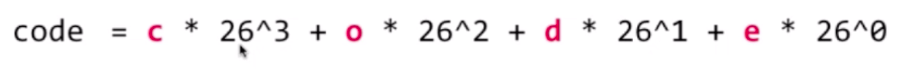#### hash冲突——链地址法


public class Hash_Table<K, V> {
private TreeMap<K, V>[] hashtable;
private int M;
private int size;

public Hash_Table(int M) {
this.M = M;
size = 0;
hashtable = new TreeMap[M];
for (int i = 0; i < hashtable.length; i++) {
hashtable[i] = new TreeMap<>();
}
}

public Hash_Table() {
this(97);
}

private int hash(K key) {
return (key.hashCode() & 0x7fffffff) % M;
}

public int getSize() {
return size;
}

public void add(K key, V value) {
if (hashtable[hash(key)].containsKey(key)) {
hashtable[hash(key)].put(key, value);
} else {
hashtable[hash(key)].put(key, value);
size++;
}
}

public V remove(K key){
TreeMap<K, V> treeMap = hashtable[hash(key)];
V ret = null;
if (treeMap.containsKey(key)){
ret = treeMap.remove(key);
size--;
}
return ret;
}

public void set(K key, V value){
TreeMap<K, V> treeMap = hashtable[hash(key)];
if (!treeMap.containsKey(key)){
throw new IllegalArgumentException("no element!");
}else {
treeMap.put(key, value);
}
}

public boolean contains(K key){
return hashtable[hash(key)].containsKey(key);
}

public V get(K key){
return hashtable[hash(key)].get(key);
}
}



    private void resize(int capacity){
TreeMap<K, V>[] newHashTable = new TreeMap[capacity];
for (int i = 0; i < capacity; i++) {
newHashTable[i] = new TreeMap<>();
}
int oldM = this.M;
this.M = capacity;
for (int i = 0; i < oldM; i++) {
TreeMap<K, V> treeMap = hashtable[i];
for (K key : treeMap.keySet()){
newHashTable[hash(key)].put(key, treeMap.get(key));
}
}

this.hashtable = newHashTable;
}



#### 最后附上所有GitHub代码：https://github.com/GreenArrow2017/DataStructure_Java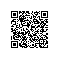使用钉钉扫一扫加入圈子
+ 订阅